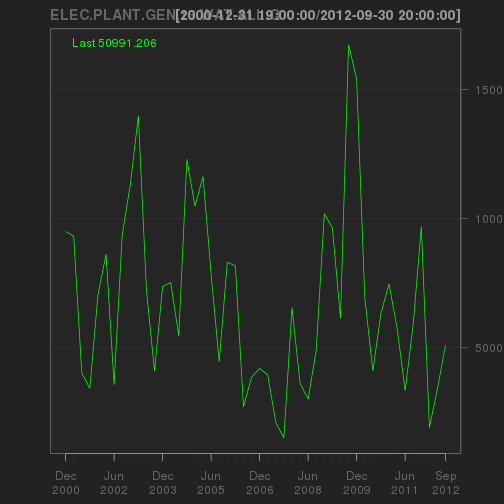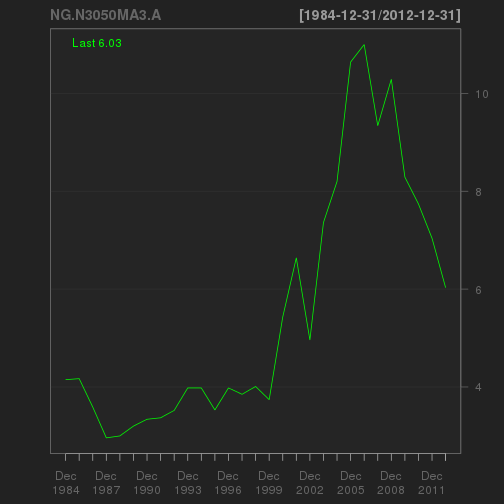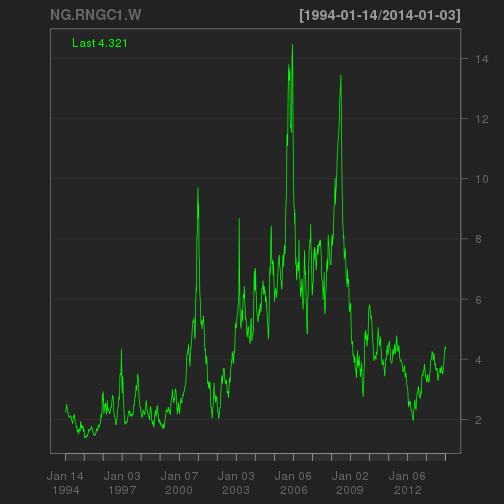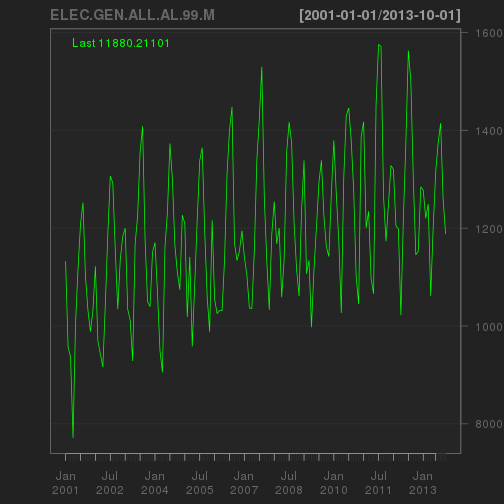# EPA's Proposed Carbon Pollution Reductions by State

The EPA has recently announced a proposal to reduce carbon pollution.  Specifically the EPA has set state targets for emission rates (the number of pounds of carbon emissions per megawatt hour of electricity produced).  A summary of the percent reduction in proposed emission rate by state is below.The proposed reductions range from a maximum percent reduction of 71.82% in Washington state, to a minimum percent reduction of 10.58% in North Dakota.

Of course, looking at percent reductions loses scale -- which is paramount in this case.  If we look at total yearly proposed carbon reductions (in million metric tons) we see the largest reduction is by far in Texas (87 million metric tons per year) with Florida and Pennsylvania following.# Baker Hughes (BHI) to Disclose All Chemical it Uses in Fracking

See here for the original Bloomberg article and Baker Hughes' disclosure policy here. I think this is a good move by Baker Hughes -- the economic benefit from nondisclosure is probably less than the costs it incurres.

Further, I think this gives Baker Hughes an advantage over its larger rivals (Schlumberger and Halliburton) as natural gas drilling activity is poised to pick up. Baker Hughes stock price over the last 5 years is below (it pays a $0.68 per year dividend also).# Markov Switching Volatilty in Weekly Natural Gas Price Returns (Henry Hub Spot) In a few earlier posts (here and here) I estimated the Time Varying volatility in daily natural gas futures price (NGH4) returns. This showed the distinct increase in the volatility of NGH4 in mid January 2014. Now that the natural gas market has settled I thought I would try to put the recent volatility in perspective. Pulling weekly Henry Hub spot prices from the EIA's API, I estimated the time varying weekly volatility again using a Markov Switching AR model. A plot of the state weighted annualized volatility is below. As you can see since 2010 the natural gas market has been fairly tame. However the recent volatility fits in quite normally with the pre-2010 behavior of gas prices. # Time-Varying Beta: BP versus Exxon In a recent presentation I had a plot (see below) of a Kalman filtered estimate of BP's time-varying beta coefficient (from a CAPM type market model). The plot clearly shows the effect of the 2010 Gulf oil spill on BP's beta -- it jumps up above 2.5. We also see BP's alpha drop below zero.Such a large effect on BP's beta coefficient is understandable given what its stock price was doing at the time.This prompted some in the audience to wonder how large the effect was on Exxon's beta coefficient around the time of the 1989 Valdez spill. Kalman filtered estimates of Exxon's alpha and beta around the time are below.As you can see, there is surprisingly little effect on Exxon's beta. To try and explain why I charted the stock price over the period (below) and found there was little effect on Exxon's stock. The only decline of note in Exxon's stock was due to the 1987 crash.This explains why Exxon's beta coefficient was unaffected by the spill. However, I am not sure why there was such a large effect on BP's stock price, and no measurable effect on Exxon's. I may take a look into it, and update this post later. # More on Time-Varying Volatility in NGH4 It looks like incorporating the last 12 months of daily NGH4 prices helps bring the recent volatility into focus. Estimating the Markov regime-switching AR model over the last 12 months (on daily log returns) affords: $r_{ng,t} = \begin{cases} 0.0006 -0.0429r_{ng,t-1}+ e_{S1,t}, \:\:\:\:\:\:\:\: e_{S1} \sim N(0,0.0122) \\ 0.0040+0.55185r_{ng,t-1}+e_{S2,t}, \:\:\:\:\:\:\: e_{S2} \sim N(0,0.0309) \end{cases}$ with the transition matrix: $P=\left[ \begin{array} 0.92 \;\;&\;\; 0.50 \\ { } & { } \\ 0.08 \;\;&\;\; 0.50 \end{array}\right]$ This gives annualized volatilities of 19.47% in the low vol state, and 49.08% in the high vol state. Weighting these volatilities by filtered state probability gives:and NGH4 over the same period is:# Markov Regime-Switching Volatility in Natural Gas Futures (NGH4) Natural gas futures have been volatile this past January and February. Over the next month I plan to look into the nature and determinants of the volatility (cold weather and relatively low storage volumes likely accounting for the lion's share). AS a first step, I have estimated a Markov Regime-Switching AR model on March NYMEX Natural Gas (NGH4) log returns. The AR results are: $r_{ng,t} = \begin{cases} 0.0014 + 0.1311r_{ng,t-1}+ e_{S1,t}, \:\:\:\:\:\:\:\: e_{S1} \sim N(0,0.0323) \\ 0.0056+0.3405r_{ng,t-1}+e_{S2,t}, \:\:\:\:\:\:\: e_{S2} \sim N(0,0.0050) \end{cases}$ with the transition matrix: $P=\left[ \begin{array} 0.78 \;\;&\;\; 0.51 \\ { } & { } \\ 0.22 \;\;&\;\; 0.49 \end{array}\right]$ The results indicate a high volatility and low volatility state with a 51% and 7.9% annualized volatility respectively. Weighting these unconditional state volatilities by the filtered state probability (chart below) gives an estimate of the time-varying volatility in NGH4.The most recent estimate is a 38% annualized volatility. A chart of NGH4 is below for the same time period.# Quarterly and Annual R Functions to Pull Time Series from the EIA's API Below are functions to pull quarterly and annual time series from the EIA's API. The functions return time series of class xts. You'll need the following libraries loaded (quantmod is just for charting): library(XML) library(plyr) library(xts) library(quantmod)  Then make sure you have your key: key <- "[insert your key in quotes]"  If you don't yet have a key, see the earlier post on the function for getting categories. The function for quarterly data is: getQEIA <- function(ID, key) { ID <- unlist(strsplit(ID, ";")) key <- unlist(strsplit(key, ";")) url <- paste("http://api.eia.gov/series?series_id=", ID, "&api_key=", key, "&out=xml", sep = "") doc <- xmlParse(file = url, isURL = TRUE) df <- xmlToDataFrame(nodes = getNodeSet(doc, "//data/row")) df <- arrange(df, df$date)

date <- as.yearqtr(df$date) values <- as.numeric(levels(df[, -1]))[df[, -1]] xts_data <- xts(values, order.by = date) names(xts_data) <- sapply(strsplit(ID, "-"), paste, collapse = ".") assign(sapply(strsplit(ID, "-"), paste, collapse = "."), xts_data, envir = .GlobalEnv) }  The function for annual data is: getAnnEIA <- function(ID, key) { ID <- unlist(strsplit(ID, ";")) key <- unlist(strsplit(key, ";")) url <- paste("http://api.eia.gov/series?series_id=", ID, "&api_key=", key, "&out=xml", sep = "") doc <- xmlParse(file = url, isURL = TRUE) df <- xmlToDataFrame(nodes = getNodeSet(doc, "//data/row")) df <- arrange(df, df$date)
date <- as.Date(paste(as.character(levels(df[, 1]))[df[, 1]], "-12-31",
sep = ""), "%Y-%m-%d")
values <- as.numeric(levels(df[, -1]))[df[, -1]]

xts_data <- xts(values, order.by = date)
names(xts_data) <- sapply(strsplit(ID, "-"), paste, collapse = ".")

assign(sapply(strsplit(ID, "-"), paste, collapse = "."), xts_data, envir = .GlobalEnv)
}


Then, to use the function type:

getQEIA(ID = "ELEC.PLANT.GEN.13-WAT-ALL.Q", key = key)


Which will return an time series object of class xts named ELEC.PLANT.GEN.13.WAT.ALL.Q to the global environment (note the substitution of . for -). This time series is the number of megawatt hours of electricity produced by the Jordan Hydroelectric Dam per quarter. The time series is ready to be used by quantmod:

chartSeries(ELEC.PLANT.GEN.13.WAT.ALL.Q)Similarly for annual data:

getAnnEIA("NG.N3050MA3.A", key = key)


This is the annual natural gas citygate price in Massachusetts.

chartSeries(NG.N3050MA3.A)Note all of these functions pull the full time series history. However, since the resulting time series object is of class xts, it is very easy to return a subset of the time series. For example, if you just want the gas prices from 2005 onward you can use:

NG.N3050MA3.A["2005/"]

##            NG.N3050MA3.A
## 2005-12-31         10.64
## 2006-12-31         11.00
## 2007-12-31          9.34
## 2008-12-31         10.29
## 2009-12-31          8.29
## 2010-12-31          7.74
## 2011-12-31          7.04
## 2012-12-31          6.03


For more information on how to use xts series use ?xts in R.

# R Functions to get Daily, Weekly, or Monthly Time Series from the EIA's API

Below are functions to pull daily, weekly, and monthly data from the EIA. The function returns a time series of class xts. The next step is to roll all of these functions into one getEIA() function which will handle any time series frequency.

You'll need the following libraries loaded (quantmod is just for charting):

library(XML)
library(plyr)
library(xts)
library(quantmod)


Then make sure you have your key:

key <- "[insert your key in quotes]"


If you don't yet have a key, see the earlier post on the function for getting categories.

The function for daily or weekly time series is:

getWDEIA <- function(ID, key) {

ID <- unlist(strsplit(ID, ";"))
key <- unlist(strsplit(key, ";"))

url <- paste("http://api.eia.gov/series?series_id=", ID, "&api_key=", key,
"&out=xml", sep = "")

doc <- xmlParse(file = url, isURL = TRUE)

df <- xmlToDataFrame(nodes = getNodeSet(doc, "//data/row"))

df <- arrange(df, df$date) date <- as.Date(df$date, "%Y%m%d")
values <- as.numeric(levels(df[, -1]))[df[, -1]]

xts_data <- xts(values, order.by = date)
names(xts_data) <- sapply(strsplit(ID, "-"), paste, collapse = ".")

assign(sapply(strsplit(ID, "-"), paste, collapse = "."), xts_data, envir = .GlobalEnv)
}


The function for monthly data is:

getMonEIA <- function(ID, key) {

ID <- unlist(strsplit(ID, ";"))
key <- unlist(strsplit(key, ";"))

url <- paste("http://api.eia.gov/series?series_id=", ID, "&api_key=", key,
"&out=xml", sep = "")

doc <- xmlParse(file = url, isURL = TRUE)

df <- xmlToDataFrame(nodes = getNodeSet(doc, "//data/row"))

df <- arrange(df, df\$date)

date <- as.Date(paste(as.character(levels(df[, 1]))[df[, 1]], "01", sep = ""),
"%Y%m%d")
values <- as.numeric(levels(df[, -1]))[df[, -1]]

xts_data <- xts(values, order.by = date)
names(xts_data) <- sapply(strsplit(ID, "-"), paste, collapse = ".")

assign(sapply(strsplit(ID, "-"), paste, collapse = "."), xts_data, envir = .GlobalEnv)
}


Then you can use the function like:

getWDEIA(ID = "NG.RNGC1.W", key = key)


Which will return an time series object of class xts named NG.RNGC1.W to the global environment. The time series is ready to be used by quantmod:

chartSeries(NG.RNGC1.W)Similarly for monthly data:

getMonEIA("ELEC.GEN.ALL-AL-99.M", key = key)


Now note, R will not allow the resulting object to be named ELEC.GEN.ALL-AL-99.M because of the “-” signs, so the function will automatically change the “-” to “.”. The object is thus named ELEC.GEN.ALL.AL.99.M and is assinged to the global environment.

chartSeries(ELEC.GEN.ALL.AL.99.M)I'll post the quarterly and yearly functions soon. Enjoy!

# R Functions to Interact with the EIA's Application Programming Interface (API)

The Energy Information Administration at the US Department of Energy has made a great deal of data available through an API. The data sets include 408,000 electricity series, 115,052 petroleum series, and 11,989 natural gas series. There are also 30,000 State Energy Data System series. The series can be browsed here: http://www.eia.gov/beta/api/qb.cfm

I have created a couple of R functions which will allow you to browse the data sets from with R, and download data directly into R as an object of class xts. Following is the function to browse the categories and find series IDs form within R – I'll post the functions which extract the data shortly. These functions are works in progress.

To use the functions you'll need to request a key from the EIA here: http://www.eia.gov/beta/api/register.cfm

You will need to have the following libraries XML and plyr libraries loaded.

library(XML)
library(plyr)


The function is:

getCatEIA <- function(key, cat=999999999){

key <- unlist(strsplit(key, ";"))

ifelse(cat==999999999,
url <- paste("http://api.eia.gov/category?api_key=", key, "&out=xml", sep="" ),

url <- paste("http://api.eia.gov/category?api_key=", key, "&category_id=", cat, "&out=xml", sep="" )
)

doc <- xmlParse(file=url, isURL=TRUE)

print("########Parent Category########")
tryCatch(print(xmlToDataFrame(nodes = getNodeSet(doc, "//category/parent_category_id"))), warning=function(w) FALSE, error=function(w) FALSE)

print("########Sub-Categories########")
print(xmlToDataFrame(nodes = getNodeSet(doc, "//childcategories/row")))

print("########Series IDs########")
print(xmlToDataFrame(nodes = getNodeSet(doc, "///childseries/row")))
}


To use the function you pass your key, and the optional category ID. If you leave the category ID blank the function will return the top categories. For example:

key <- "[your key here]"
cat <- 40827


Then:

getCatEIA(key, cat)


returns

 "########Parent Category########"
text
1 40203
 "########Sub-Categories########"
category_id           name
1       40828           Real
2       40829 Current-dollar
 "########Series IDs########"
data frame with 0 columns and 0 rows


The result shows you the parent and sub categories. Note there are no series in the category. The series IDs are the identifiers for the actual data.

To see the root (top level directory) use:

getCatEIA(key)


which returns:

 "########Parent Category########"
 "########Sub-Categories########"
category_id                            name
1       40203 State Energy Data System (SEDS)
2           0                     Electricity
3      456170                     Natural Gas
4      229973                       Petroleum
 "########Series IDs########"
data frame with 0 columns and 0 rows


To see series IDs we can choose:

getCatEIA(key, cat=40828)


which returns

 "########Parent Category########"
text
1 40827
 "########Sub-Categories########"
data frame with 0 columns and 0 rows
 "########Series IDs########"
series_id                                              name f
1  SEDS.GDPRX.OH.A                 Real gross domestic product, Ohio A
2  SEDS.GDPRX.MT.A              Real gross domestic product, Montana A
3  SEDS.GDPRX.NC.A       Real gross domestic product, North Carolina A

units               updated
Million chained (2005) dollars 26-JUN-13 06.34.27 PM
Million chained (2005) dollars 26-JUN-13 06.34.27 PM
Million chained (2005) dollars 26-JUN-13 06.34.27 PM
< there are 52 total series here:  showing 3 for space>


You can use these series IDs (together with function I'll post shortly) to pull the data.